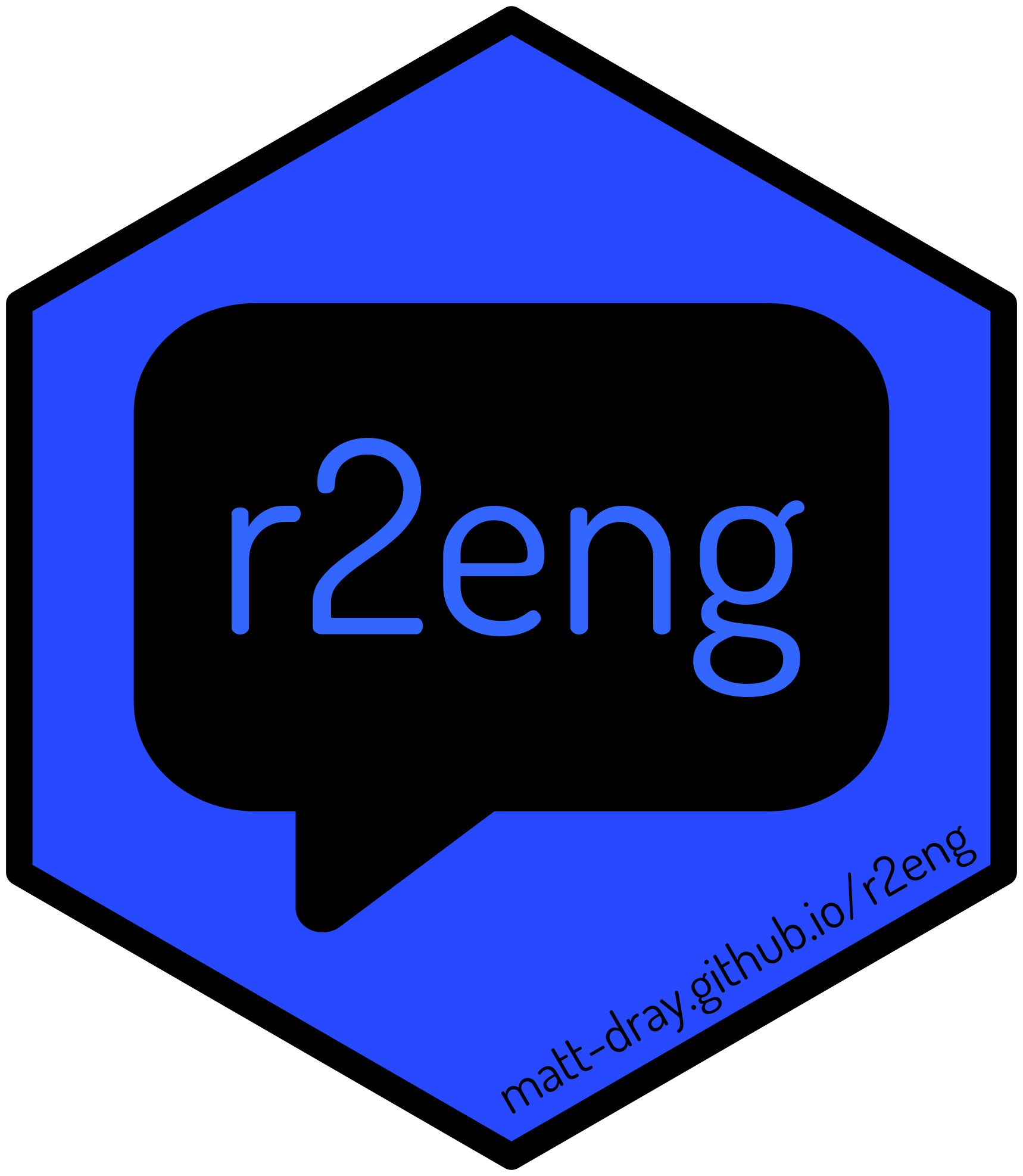ɑː ˈtuː /eng/

Make R speakable!

The goal of {r2eng} (as in ‘R to English’) is to take an R expression and ‘translate’ it to an English sentence.

The package is intended to:

• improve communication between teachers and learners
• make R discussions in English more accessible to non-English speakers
• provide an extra audio evaluation tool for users who are blind or have low vision
• be of interest to any R user that’s curious about how R expressions might be vocalised

The project was inspired by Amelia McNamara‘s useR! 2020 talk called ’Speaking R’ (YouTube, slides).

This project is a work in progress and highly opinionated. Contributions are welcome, but please see the Code of Conduct.

## Installation

You can install the development version of {r2eng} from GitHub with:

remotes::install_github("matt-dray/r2eng")

This package depends on {lintr}, {purrr} and {rlang}.

## Examples

The main function in the package is translate(). It uses non-standard evaluation, so you pass it a bare R expression like this:

r2eng::translate(variable <- 1, speak = TRUE)
# Original expression: variable <- 1
# English expression: variable gets 1

Set speak = TRUE for a system call that will read the English sentence out loud (macOS only).

A more complex example:

obj <- r2eng::translate(
hello <- c(TRUE, FALSE, 'banana' %in% c('apple', 'orange')),
speak = FALSE
)

obj
# Original expression: hello <- c(TRUE, FALSE, "banana" %in% c("apple", "orange"))
# English expression: hello gets a vector of open paren TRUE , FALSE , string "banana" matches a vector of open paren string "apple" , string "orange" close paren close paren

### Methods

An r2eng object has the methods speak and evaluate.

Use speak to launch a system call that will vocalise the translated English sentence for the given R expression (macOS only).

r2eng::speak(obj)

Use evaluate to evaluate the expression.

r2eng::evaluate(obj, speak = FALSE)
hello
#   TRUE FALSE FALSE

Use print to print the R expression and English sentence.

print(obj)
# Original expression: hello <- c(TRUE, FALSE, "banana" %in% c("apple", "orange"))
# English expression: hello gets a vector of open paren TRUE , FALSE , string "banana" matches a vector of open paren string "apple" , string "orange" close paren close paren

From your r2eng object you can access the original R expression (r_expression), English translation (eng_expression), quoted expression (quoted_expression). You can also access the parse tree output via {lintr} (translation_map):

head(obj\$translation_map)
#                   token  text         eng
# 40                 expr
# 1                SYMBOL hello       hello
# 3                  expr
# 2           LEFT_ASSIGN    <-        gets
# 38                 expr
# 4  SYMBOL_FUNCTION_CALL     c a vector of

### Further examples

Here’s an example using the pipe (%>%) and two types of ‘equals’:

library(magrittr)
r2eng::translate(
mtcars %>% filter(mpg > 22) %>% mutate(gear4 = gear == 4),
speak = FALSE
)
# Original expression: mtcars %>% filter(mpg > 22) %>% mutate(gear4 = gear == 4)
# English expression: mtcars then filter of open paren mpg is greater than 22 close paren then mutate of open paren gear4 = gear double equals 4 close paren

This example uses the ‘plus’ constructor from {ggplot2}:

r2eng::translate(
ggplot(diamonds, aes(x=carat, y=price, color=cut)) + geom_point() + geom_smooth(),
speak = FALSE
)
# Original expression: ggplot(diamonds, aes(x = carat, y = price, color = cut)) + geom_point() +
#  Original expression:     geom_smooth()
# English expression: ggplot of open paren diamonds , aes of open paren x = carat , y = price , color = cut close paren close paren plus geom_point of open paren close paren plus geom_smooth of open paren close paren

This example shows what happens when you pass vectors:

r2eng::translate(
plot(x = c(1, 2, 3), y = c(5, 6, 7)),
speak = FALSE
)
# Original expression: plot(x = c(1, 2, 3), y = c(5, 6, 7))
# English expression: plot of open paren x = a vector of open paren 1 , 2 , 3 close paren , y = a vector of open paren 5 , 6 , 7 close paren close paren

### Passing a string

The translate() function understands the meaning of = when used for assignment versus specifying arguments, but feeding an expression such as x = c(1, 2, 3) would confuse translate() that you want to pass an argument c(1, 2, 3) to the parameter x.

This is because translate() uses non-standard evaluation.

In such cases, you must use translate_string() instead:

r2eng::translate_string("x = c(1, 2, 3)", speak = FALSE)
# Original expression: x = c(1, 2, 3)
# English expression: x gets a vector of open paren 1 , 2 , 3 close paren

Another exceptional case for translate_string() is when piping and expression:

"non_english <- c('ceci n est pas une pipe', 'Ich bin ein Berliner', '我其實唔識講廣東話')" %>%
r2eng::translate_string(speak = FALSE)
# Original expression: non_english <- c("ceci n est pas une pipe", "Ich bin ein Berliner",
#  Original expression:     "我其實唔識講廣東話")
# English expression: non_english gets a vector of open paren string "ceci n est pas une pipe" , string "Ich bin ein Berliner" , string "我其實唔識講廣東話" close paren

Installing this package also installs an RStudio addin.

Select an R expression in the editor and then under ‘Addins’, go to ‘Speak R Expression In English’ under ‘R2ENG’. The selected text will be spoken by your computer.

You could bind this addin to a keyboard shortcut in RStudio by going to ‘Tools’, then ‘Modify Keyboard Shortcuts…’. Perhaps Cmd + Shift + V.

As with the rest of this package, vocalisation is only possible for macOS and with speakers.

Another Addin function is also available, ‘Print R Expression In English’, which prints a selected R expression and its English translation

## Contributions and Code of Conduct

Contributions are welcome from everyone. Please first add an issue if a relevant one one doesn’t already exist.

Please note that the {r2eng} project is released with a Contributor Code of Conduct. By contributing to this project, you agree to abide by its terms.